# Unit 3 Informed Search Strategies.pptx

31. May 2023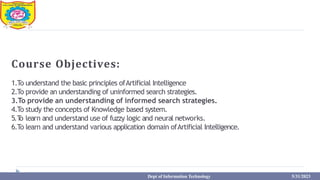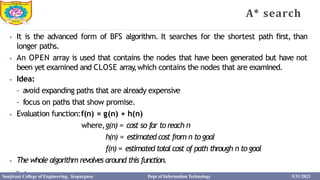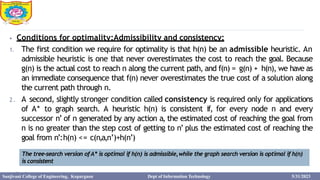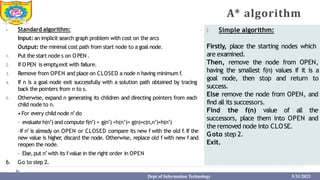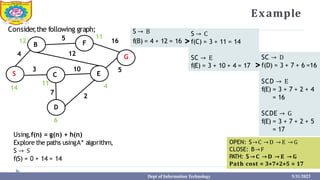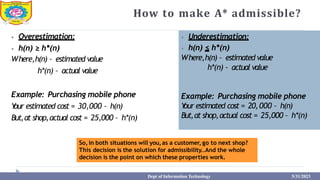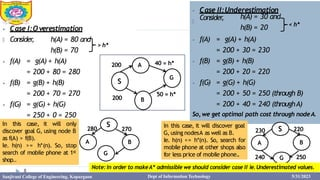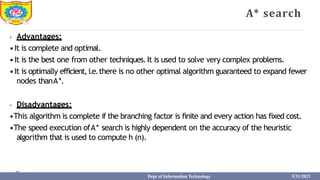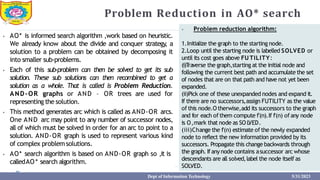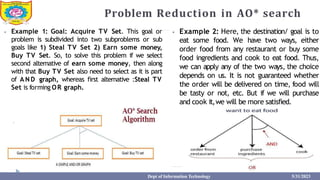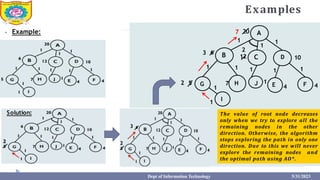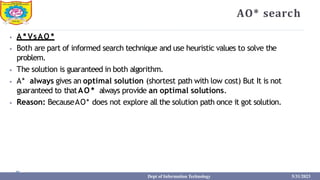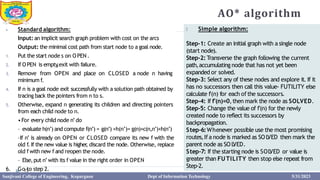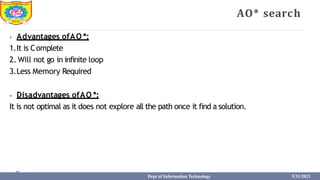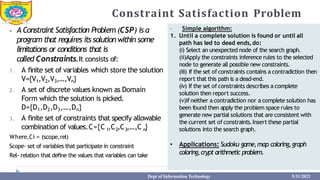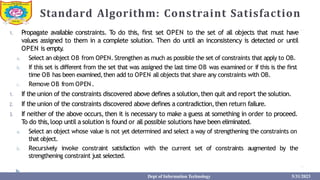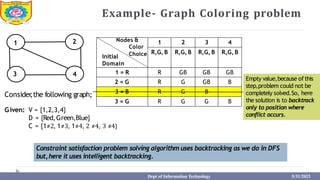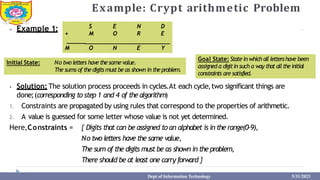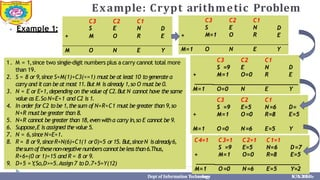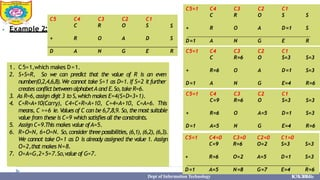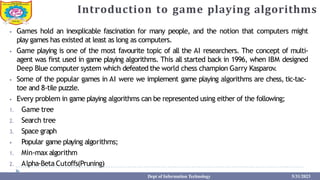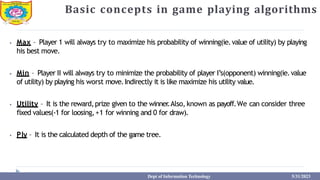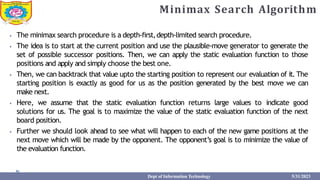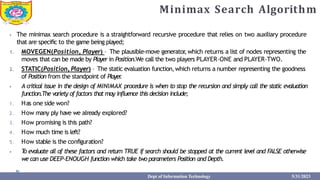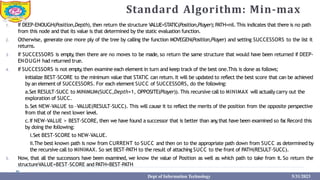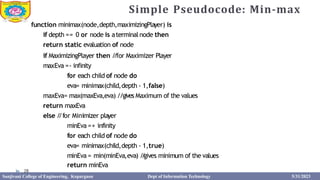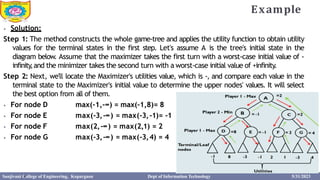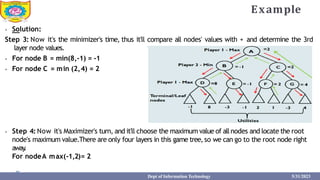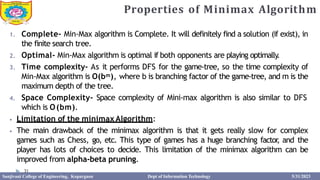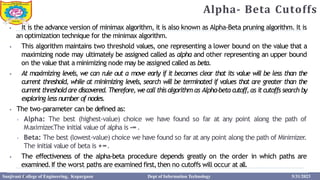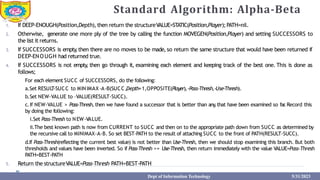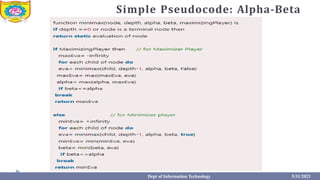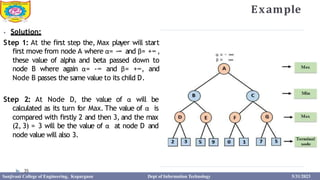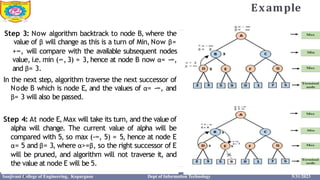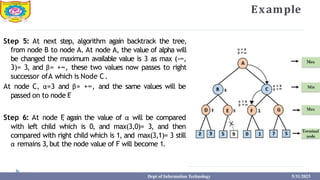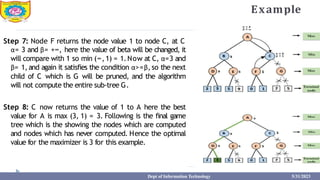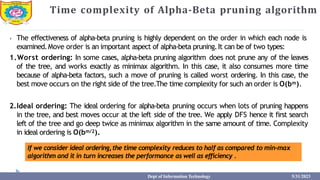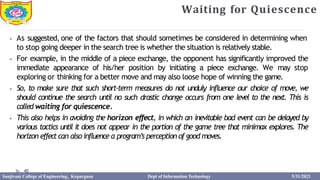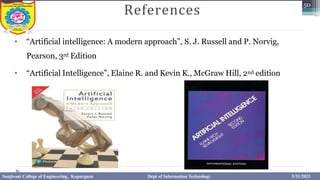1 von 42

### Unit 3 Informed Search Strategies.pptx

• 1. Sanjivani Rural Education Society’s Sanjivani College of Engineering, Kopargaon-423603 (AnAutonomous Institute Affiliated to Savitribai Phule Pune University, Pune) NAAC ‘A’GradeAccredited, ISO 9001:2015 Certified Department of Information Technology (NBA Accredited) Department:- Information Technology Name of Subject:- Artificial Intelligence Class:- TYIT Subject Code:- IT313 Sanjivani College of Engineering, Kopargaon Dept of Information Technology 5/31/2023
• 2. Course Objectives: 1.To understand the basic principles ofArtificial Intelligence 2.To provide an understanding of uninformed search strategies. 3.To provide an understanding of informed search strategies. 4.To study the concepts of Knowledge based system. 5.T o learn and understand use of fuzzy logic and neural networks. 6.To learn and understand various application domain ofArtificial Intelligence. Dept of Information Technology 5/31/2023
• 3. A* search 3 ⦁ It is the advanced form of BFS algorithm. It searches for the shortest path first, than longer paths. ⦁ An OPEN array is used that contains the nodes that have been generated but have not been yet examined and CLOSE array ,which contains the nodes that are examined. ⦁ Idea: – avoid expanding paths that are already expensive – focus on paths that show promise. ⦁ Evaluation function:f(n) = g(n) + h(n) where,g(n) = cost so far toreach n h(n) = estimated cost from n togoal f(n) = estimated total cost of path through n togoal ⦁ The whole algorithm revolves around this function. Sanjivani College of Engineering, Kopargaon Dept of Information Technology 5/31/2023
• 4. 4 ⦁ Conditions for optimality:Admissibility and consistency: 1. The first condition we require for optimality is that h(n) be an admissible heuristic. An admissible heuristic is one that never overestimates the cost to reach the goal. Because g(n) is the actual cost to reach n along the current path, and f(n) = g(n) + h(n), we have as an immediate consequence that f(n) never overestimates the true cost of a solution along the current path through n. 2. A second, slightly stronger condition called consistency is required only for applications of A* to graph search. A heuristic h(n) is consistent if, for every node n and every successor n’ of n generated by any action a, the estimated cost of reaching the goal from n is no greater than the step cost of getting to n’ plus the estimated cost of reaching the goal from n’:h(n) <= c(n,a,n’)+h(n’) The tree-search version ofA* is optimal if h(n) is admissible,while the graph search version is optimal if h(n) is consistent Sanjivani College of Engineering, Kopargaon Dept of Information Technology 5/31/2023
• 5. A* algorithm ⦁ Standard algorithm: Input: an implicit search graph problem with cost on the arcs Output: the minimal cost path from start node to a goal node. 1. Put the start node s on OPEN. 2. If OPEN is empty ,exit with failure. 3. Remove from OPEN and place on CLOSED a node n having minimum f. 4. If n is a goal node exit successfully with a solution path obtained by tracing back the pointers from n to s. 5. Otherwise, expand n generating its children and directing pointers from each child node to n. •For every child node n’do – evaluate h(n’) and compute f(n’) = g(n’) +h(n’)= g(n)+c(n,n’)+h(n’) –If n’ is already on OPEN or CLOSED compare its new f with the old f. If the new value is higher , discard the node. Otherwise, replace old f with new f and reopen the node. – Else,put n’with its f value in the right order in OPEN 6. Go to step 2. 🞂 Simple algorithm: Firstly, place the starting nodes which are examined. Then, remove the node from OPEN, having the smallest f(n) values if it is a goal node, then stop and return to success. Else remove the node from OPEN, and find all its successors. Find the f(n) value of all the successors, place them into OPEN and the removed node into CLOSE. Goto step 2. Exit. Dept of Information Technology 5/31/2023
• 6. Example Consider ,the following graph; E F C B G 10 2 3 12 5 5 7 16 S 14 OPEN: S→C →D → E → G CLOSE: B→F PATH: S → C →D → E → G Path cost = 3+7+2+5 = 17 11 D 6 12 4 11 4 Using,f(n) = g(n) + h(n) Explore the paths usingA* algorithm, S → S f(S) = 0 + 14 = 14 S → C f(C) = 3 + 11 = 14 SC → E S → B f(B) = 4 + 12 = 16 > SC → D f(D) = 3 + 7 + 6 =16 Dept of Information Technology 5/31/2023 SCD → E f(E) = 3 + 7 + 2 + 4 = 16 SCDE → G f(E) = 3 + 7 + 2 + 5 = 17 f(E) = 3 + 10 + 4 = 17 >
• 7. How to make A* admissible? ⦁ Overestimation: ⦁ h(n) ≥ h*(n) Where,h(n) – estimated value h*(n) – actual value Example: Purchasing mobile phone Y our estimated cost = 30,000 – h(n) But,at shop,actual cost = 25,000 – h*(n) ⦁ Underestimation: ⦁ h(n) ≤ h*(n) Where,h(n) – estimated value h*(n) – actual value Dept of Information Technology 5/31/2023 Example: Purchasing mobile phone Y our estimated cost = 20,000 – h(n) But,at shop,actual cost = 25,000 – h*(n) So, in both situations will you,as a customer, go to next shop? This decision is the solution for admissibility..And the whole decision is the point on which these properties work.
• 8. ⦁ Case I:Overestimation 🞂 Consider , h(A) = 80 and h(B) = 70 ⦁ f(A) ⦁ f(B) ⦁ f(G) = g(A) + h(A) = 200 + 80 = 280 = g(B) + h(B) = 200 + 70 = 270 = g(G) + h(G) = 250 + 0 = 250 S G A B 200 200 40 = h* 50 = h* > h* ⦁ Case II:Underestimation 🞂 Consider , h(A) = 30 and h(B) = 20 ⦁ f(A) ⦁ f(B) ⦁ f(G) = g(A) + h(A) = 200 + 30 = 230 = g(B) + h(B) = 200 + 20 = 220 = g(G) + h(G) = 200 + 50 = 250 (through B) = 200 + 40 = 240 (throughA) So, we get optimal path cost through nodeA. < h* S A B G 280 270 B G 230 A S 220 240 250 In this case, it will only discover goal G, using node B as f(A) > f(B). Ie. h(n) >= h*(n). So, stop search of mobile phone at 1st shop.. In this case, it will discover goal G, using nodesA as well as B. Ie. h(n) <= h*(n). So, search for mobile phone at other shops also for less price of mobile phone.. Note:In order to makeA* admissible we should consider case II ie.Underestimated values. 8 Sanjivani College of Engineering, Kopargaon Dept of Information Technology 5/31/2023
• 9. A* search ⦁ Advantages: •It is complete and optimal. •It is the best one from other techniques.It is used to solve very complex problems. •It is optimally efficient,i.e.there is no other optimal algorithm guaranteed to expand fewer nodes thanA*. ⦁ Disadvantages: •This algorithm is complete if the branching factor is finite and every action has fixed cost. •The speed execution ofA* search is highly dependent on the accuracy of the heuristic algorithm that is used to compute h (n). Dept of Information Technology 5/31/2023
• 10. AO* search Example: B D G H F E 1. A → BC (AND arc) f(A1) = 6+12+1+1 = 20 2. A → D f(A2) = 10+1 = 11 3. f(A1) > f(A2). So, explore path through node D. 4. D → EF f(D) = 4+4+2 = 10 5. Implementation of AO* algorithm will only explore path for the above graph through node D. Though the possibility of getting optimal solution can be in other direction. ⦁ AO* Algorithm on problem basically based decompositon (Breakdown problem into small pieces) ⦁ When a problem can be divided into a set of sub problems, where each sub problem can be solved separately and a combination of these will be a solution, A N D -OR graphs or A N D - OR trees are used for representing the solution. ⦁ The decomposition of the problem or problemreduction generatesAND arcs. ⦁ The main difference between A* and AO* algorithm is, AO* does not explore all the solution paths once it got a solution. 1 1 A 1 C 1 1 6 5 12 1 10 1 4 4 7 Solution: Dept of Information Technology 5/31/2023
• 11. Problem Reduction in AO* search ⦁ AO* is informed search algorithm ,work based on heuristic. We already know about the divide and conquer strategy, a solution to a problem can be obtained by decomposing it into smaller sub-problems. ⦁ Each of this sub-problem can then be solved to get its sub solution. These sub solutions can then recombined to get a solution as a whole. That is called is Problem Reduction. AND-OR graphs or AND – OR trees are used for representing the solution. ⦁ This method generates arc which is called as AND-OR arcs. One AND arc may point to any number of successor nodes, all of which must be solved in order for an arc to point to a solution. AND-OR graph is used to represent various kind of complex problemsolutions. ⦁ AO* search algorithm is based on AND-OR graph so ,it is calledAO* search algorithm. ⦁ Problem reduction algorithm: 1.Initialize the graph to the starting node. 2.Loop until the starting node is labelled SOLVED or until its cost goes above FU TILITY: (i)T raverse the graph,starting at the initial node and following the current best path and accumulate the set of nodes that are on that path and have not yet been expanded. (ii)Pick one of these unexpanded nodes and expand it. If there are no successors,assign FUTILITY as the value of this node.O therwise,add its successors to the graph and for each ofthem compute f'(n).If f'(n) ofany node is O,mark that node as SO L VED. (iii)Change the f'(n) estimate of the newly expanded node to reflect the new information provided by its successors. Propagate this change backwards through the graph. Ifanynode contains asuccessor arc whose descendants are all solved,label the node itself as SOLVED. Dept of Information Technology 5/31/2023
• 12. Problem Reduction in AO* search ⦁ Example 1: Goal: Acquire TV Set. This goal or problem is subdivided into two subproblems or sub goals like 1) Steal TV Set 2) Earn some money, Buy TV Set. So, to solve this problem if we select second alternative of earn some money, then along with that Buy TV Set also need to select as it is part of A N D graph, whereas first alternative :Steal TV Set is forming OR graph. ⦁ Example 2: Here, the destination/ goal is to eat some food. We have two ways, either order food from any restaurant or buy some food ingredients and cook to eat food. Thus, we can apply any of the two ways, the choice depends on us. It is not guaranteed whether the order will be delivered on time, food will be tasty or not, etc. But if we will purchase and cook it,we will be more satisfied. Dept of Information Technology 5/31/2023
• 13. Examples B D G F ⦁ Example: 1 1 A 1 C 1 1 10 1 4 4 7 H J 1 I 1 1 1 E 2 5 3 6 7 20 1 2 12 Solution: The value of root node decreases only when we try to explore all the remaining nodes in the other direction. Otherwise, the algorithm stops exploring the path in only one direction. Due to this we will never explore the remaining nodes and the optimal path using AO*. Dept of Information Technology 5/31/2023
• 14. AO* search ⦁ A * VsAO * ⦁ Both are part of informed search technique and use heuristic values to solve the problem. ⦁ The solution is guaranteed in both algorithm. ⦁ A* always gives an optimal solution (shortest path with low cost) But It is not guaranteed to thatAO * always provide an optimal solutions. ⦁ Reason: BecauseAO* does not explore all the solution path once it got solution. Dept of Information Technology 5/31/2023
• 15. AO* algorithm ⦁ Standard algorithm: Input: an implicit search graph problem with cost on the arcs Output: the minimal cost path from start node to a goal node. 1. Put the start node s on OPEN. 2. If OPEN is empty ,exit with failure. 3. Remove from OPEN and place on CLOSED a node n having minimum f. 4. If n is a goal node exit successfully with a solution path obtained by tracing back the pointers from n to s. 5. Otherwise, expand n generating its children and directing pointers from each child node to n. •For every child node n’do – evaluate h(n’) and compute f(n’) = g(n’) +h(n’)= g(n)+c(n,n’)+h(n’) –If n’ is already on OPEN or CLOSED compare its new f with the old f.If the new value is higher , discard the node. Otherwise, replace old f with new f and reopen the node. – Else,put n’with its f value in the right order in OPEN 6. Go 15 to step 2. 🞂 Simple algorithm: Step-1: Create an initial graph with a single node (start node). Step-2: Transverse the graph following the current path,accumulating node that has not yet been expanded or solved. Step-3: Select any of these nodes and explore it. If it has no successors then call this value- FUTILITY else calculate f'(n) for each ofthe successors. Step-4: If f'(n)=0,then mark the node as SOLVED. Step-5: Change the value of f'(n) for the newly created node to reflect its successors by backpropagation. Step-6:Whenever possible use the most promising routes,Ifa node is marked as SOL VED then mark the parent node as SO L VED. Step-7: If the starting node is SOL VED or value is greater than FUTILITY then stop else repeat from Step-2. Sanjivani College of Engineering, Kopargaon Dept of Information Technology 5/31/2023
• 16. AO* search ⦁ Advantages ofAO *: 1.It is Complete 2. Will not go in infinite loop 3.Less Memory Required ⦁ Disadvantages ofAO *: It is not optimal as it does not explore all the path once it find a solution. Dept of Information Technology 5/31/2023
• 17. Constraint Satisfaction Problem ⦁ AConstraint Satisfaction Problem (CSP) is a program that requires its solution within some limitations or conditions that is called Constraints.It consists of: 1. A finite set of variables which store the solution V={V1,V2,V3,…,Vn} 2. A set of discrete values known as Domain Form which the solution is picked. D={D1,D2,D3,…,Dn} 3. A finite set of constraints that specify allowable 1 2 3 n combination of values.C={C ,C ,C ,…,C } Where,Ci = (scope,rel) Scope- set of variables that participate in constraint Rel- relation that define the values that variables can take • Simple algorithm: 1. Until a complete solution is found or until all path has led to dead ends,do: (i) Select an unexpected node of the search graph. (ii)Apply the constraints inference rules to the selected node to generate all possible new constraints. (iii) If the set of constraints contains a contradiction then report that this path is a dead-end. (iv) If the set of constraints describes a complete solution then report success. (v)Ifneither a contradiction nor a complete solution has been found then apply the problem space rules to generate new partial solutions that are consistent with the current set of constraints.Insert these partial solutions into the search graph. • Applications: Sudoku game,map coloring,graph coloring,crypt arithmetic problem. Dept of Information Technology 5/31/2023
• 18. Standard Algorithm: Constraint Satisfaction 1. Propagate available constraints. To do this, first set OPEN to the set of all objects that must have values assigned to them in a complete solution. Then do until an inconsistency is detected or until OPEN is empty . a. Select an object OB from OPEN. Strengthen as much as possible the set of constraints that apply to OB. b. If this set is different from the set that was assigned the last time OB was examined or if this is the first time OB has been examined,then add to OPEN all objects that share any constraints with OB. c. Remove OB from OPEN. 1. If the union of the constraints discovered above defines a solution,then quit and report the solution. 2. If the union of the constraints discovered above defines a contradiction,then return failure. 3. If neither of the above occurs, then it is necessary to make a guess at something in order to proceed. To do this,loop until a solution is found or all possible solutions have been eliminated. a. Select an object whose value is not yet determined and select a way of strengthening the constraints on that object. b. Recursively invoke constraint satisfaction with the current set of constraints augmented by the strengthening constraint just selected. Dept of Information Technology 5/31/2023
• 19. Example- Graph Coloring problem Empty value,because ofthis step,problem could not be completely solved.So, here the solution is to backtrack only to position where conflict occurs. Given: V = {1,2,3,4} D = {Red,Green,Blue} C = {1≠2, 1≠3, 1≠4, 2 ≠4, 3 ≠4} 1 2 3 4 Consider ,the following graph; Nodes & Color Initial Choice Domain 1 R,G, B 2 R,G, B 3 R,G, B 4 R,G, B 1 = R R GB GB GB 2 = G R G GB B 3 = B R G B 3 = G R G G B Constraint satisfaction problem solving algorithm uses backtracking as we do in DFS but,here it uses intelligent backtracking. Dept of Information Technology 5/31/2023
• 20. Example: Crypt arithmetic Problem ⦁ Example 1: ⦁ Solution: The solution process proceeds in cycles.At each cycle,two significant things are done;(corresponding to step 1 and 4 of the algorithm) 1. Constraints are propagated by using rules that correspond to the properties of arithmetic. 2. A value is guessed for some letter whose value is not yet determined. Here,Constraints = { Digits that can be assigned to an alphabet is in the range(0-9), No two letters have the same value, The sum of the digits must be as shown in the problem, There should be at least one carry forward } + S M E O N R D E ______ M O N E Y Initial State: Dept of Information Technology 5/31/2023 No two letters have the same value. The sums of the digits mustbe as shown in the problem. Goal State: State in which all lettershave been assigned a digitin such a way that allthe initial constraints are satisfied.
• 21. Example: Crypt arithmetic Problem ⦁ Example 1: C3 S C2 E C1 N D + M O R E M O N E Y 1. M = 1,since two single-digit numbers plus a carry cannot total more than 19. 2. S = 8 or 9,since S+M(1)+C3(<=1) must be at least 10 togenerate a carry and itcan be at most 11.But M is already 1,so O must be 0. 3. N = E or E+1,depending on the value of C2.But N cannot have the same value as E.So N=E+1 and C2 is 1. 4. In order for C2 to be 1,thesum of N+R+C1 must be greater than 9,so N+R mustbe greater than 8. 5. N+R cannot be greater than 18,even witha carry in,so E cannot be 9. 6. Suppose,E is assigned the value 5. 7. N = 6,since N=E+1. 8. R = 8 or9,sinceR+N(6)+C1(1 or0)=5 or15. But,sinceN isalready6, thesumofthesenon-negativenumberscannotbelessthan6.Thus, R+6+(0 or 1)=15 and R = 8 or 9. 9. D+5 =Y ,So,D>=5.Assign 7 to D.7+5=Y(12) + C3 S M=1 C2 E O C1 N R D E M=1 O N E Y + C3 C2 C1 S =9 E N M=1 O=0 R D E ______ M=1 O=0 N E Y C3 C2 S =9 E=5 C1 N=6 D= + M=1 O=0 R=8 E=5 M=1 O=0 N=6 E=5 Y C4=1 + C3=1 C2=1 C1=1 S =9 E=5 N=6 M=1 O=0 R=8 D=7 E=5 ______ M=1 Dept of Information Technology O=0 N=6 E=5 Y=2 K. S. Ubale 5/31/2023 Dept of Information Technology
• 22. ⦁ Example 2: C5 C4 C C3 R C2 O C1 S S + R O A D S D A N G E R 1. C5=1,which makes D=1. 2. S+S=R, So we can predict that the value of R is an even number(0,2,4,6,8).We cannot take S=1 as D=1. If S=2 it further creates conflictbetween alphabetAand E.So,take R=6. 3. As R=6,assign digit 3 to S,which makes E=4(S+D=3+1). 4. C+R=A+10(Carry), C4+C+R=A+10, C+4=A+10, C=A+6. This means, C >=6 ie. Values of C can be 6,7,8,9. So, the most suitable value from these is C=9 which satisfies all the constraints. 5. Assign C=9.This makes value ofA=5. 6. R+O=N, 6+O=N. So, consider threepossibilities,(6,1), (6,2), (6,3). We cannot take O=1 as D is already assigned the value 1. Assign O=2,that makes N=8. 7. O+A=G,2+5=7.So,value of G=7. C5=1 C4 C C3 R C2 O C1 S S + R O A D=1 S D=1 A N G E R C5=1 C4 C C3 R=6 C2 O C1 S=3 S=3 + R=6 O A D=1 S=3 D=1 A N G E=4 R=6 C5=1 C4 C=9 C3 R=6 C2 O C1 S=3 S=3 + R=6 O A=5 D=1 S=3 D=1 A=5 N G E=4 R=6 C5=1 C4=0 C=9 C3=0 R=6 C2=0 O=2 C1=0 S=3 S=3 + R=6 O=2 A=5 D=1 S=3 D=1 A=5 N=8 G=7 E=4 R=6 K. S. Ubale Dept of Information Technology 5/31/2023
• 23. Introduction to game playing algorithms ⦁ Games hold an inexplicable fascination for many people, and the notion that computers might play games has existed at least as long as computers. ⦁ Game playing is one of the most favourite topic of all the AI researchers. The concept of multi- agent was first used in game playing algorithms. This all started back in 1996, when IBM designed Deep Blue computer system which defeated the world chess champion Garry Kasparov. ⦁ Some of the popular games in AI were we implement game playing algorithms are chess, tic-tac- toe and 8-tile puzzle. ⦁ Every problem in game playing algorithms can be represented using either of the following; 1. Game tree 2. Search tree 3. Space graph ⦁ Popular game playing algorithms; 1. Min-max algorithm 2. Alpha-Beta Cutoffs(Pruning) Dept of Information Technology 5/31/2023
• 24. Basic concepts in game playing algorithms ⦁ Max – Player 1 will always try to maximize his probability of winning(ie.value of utility) by playing his best move. ⦁ Min – Player II will always try to minimize the probability of player I’s(opponent) winning(ie.value of utility) by playing his worst move.Indirectly it is like maximize his utility value. ⦁ Utility – It is the reward,prize given to the winner .Also, known as payoff.We can consider three fixed values(-1 for loosing,+1 for winning and 0 for draw). ⦁ Ply – It is the calculated depth of the game tree. Dept of Information Technology 5/31/2023
• 25. Minimax Search Algorithm ⦁ The minimax search procedure is a depth-first,depth-limited search procedure. ⦁ The idea is to start at the current position and use the plausible-move generator to generate the set of possible successor positions. Then, we can apply the static evaluation function to those positions and apply and simply choose the best one. ⦁ Then, we can backtrack that value upto the starting position to represent our evaluation of it. The starting position is exactly as good for us as the position generated by the best move we can make next. ⦁ Here, we assume that the static evaluation function returns large values to indicate good solutions for us. The goal is to maximize the value of the static evaluation function of the next board position. ⦁ Further we should look ahead to see what will happen to each of the new game positions at the next move which will be made by the opponent. The opponent’s goal is to minimize the value of the evaluation function. Dept of Information Technology 5/31/2023
• 26. Minimax Search Algorithm ⦁ The minimax search procedure is a straightforward recursive procedure that relies on two auxiliary procedure that are specific to the game being played; 1. MOVEGEN(Position, Player) – The plausible-move generator ,which returns a list of nodes representing the moves that can be made by Player in Position.We call the two players PLAYER-ONE and PLAYER-TWO. 2. STATIC(Position, Player) – The static evaluation function,which returns a number representing the goodness of Position from the standpoint of Player . ⦁ A critical issue in the design of MINIMAX procedure is when to stop the recursion and simply call the static evaluation function.The variety of factors that may influence this decision include; 1. Has one side won? 2. How many ply have we already explored? 3. How promising is this path? 4. How much time is left? 5. How stable is the configuration? ⦁ T o evaluate all of these factors and return TRUE if search should be stopped at the current level and FALSE otherwise we can use DEEP-ENOUGH function which take two parameters Position and Depth. Dept of Information Technology 5/31/2023
• 27. Standard Algorithm: Min-max 1. If DEEP-ENOUGH(Position,Depth), then return the structure VALUE=STATIC(Position,Player);PATH=nil.This indicates that there is no path from this node and that its value is that determined by the static evaluation function. 2. Otherwise, generate one more ply of the tree by calling the function MOVEGEN(Position,Player) and setting SUCCESSORS to the list it returns. 3. If SUCCESSORS is empty ,then there are no moves to be made, so return the same structure that would have been returned if DEEP- ENOUGH had returned true. 4. If SUCCESSORS is not empty ,then examine each element in turn and keep track of the best one.This is done as follows; Initialize BEST-SCORE to the minimum value that STATIC can return.It will be updated to reflect the best score that can be achieved by an element of SUCCESSORS. For each element SUCC of SUCCESSORS, do the following: a.Set RESULT-SUCC to MINIMUM(SUCC,Depth+1, OPPOSITE(Player)). This recursive call to MINIMAX will actually carry out the exploration of SUCC. b.Set NEW-VALUE to –VALUE(RESULT-SUCC). This will cause it to reflect the merits of the position from the opposite perspective from that of the next lower level. c.If NEW-VALUE > BEST-SCORE, then we have found a successor that is better than any ,that have been examined so far .Record this by doing the following: i.Set BEST-SCORE to NEW-VALUE. ii.The best known path is now from CURRENT to SUCC and then on to the appropriate path down from SUCC as determined by the recursive call to MINIMAX. So set BEST-PATH to the result of attaching SUCC to the front of PATH(RESULT-SUCC). 5. Now, that all the successors have been examined, we know the value of Position as well as which path to take from it. So return the structureVALUE=BEST-SCORE and PATH=BEST-PATH Dept of Information Technology 5/31/2023
• 28. Simple Pseudocode: Min-max function minimax(node,depth,maximizingPlayer) is if depth == 0 or node is aterminal node then return static evaluation of node if MaximizingPlayer then / /for Maximizer Player maxEva =- infinity for each child of node do eva= minimax(child,depth - 1,false) maxEva= max(maxEva,eva) //gives Maximum of the values return maxEva else / /for Minimizer player minEva =+ infinity for each child of node do eva= minimax(child,depth - 1,true) minEva = min(minEva,eva) / / gives minimum of the values return minEva 28 Sanjivani College of Engineering, Kopargaon Dept of Information Technology 5/31/2023
• 29. Example 2 9 ⦁ Solution: Step 1: The method constructs the whole game-tree and applies the utility function to obtain utility values for the terminal states in the first step. Let's assume A is the tree's initial state in the diagram below. Assume that the maximizer takes the first turn with a worst-case initial value of - infinity ,and the minimizer takes the second turn with a worst-case initial value of +infinity. Step 2: Next, we'll locate the Maximizer's utilities value, which is -, and compare each value in the terminal state to the Maximizer's initial value to determine the upper nodes' values. It will select the best option from all of them. ⦁ For node D ⦁ For node E ⦁ For node F ⦁ For node G max(-1,-∞) = max(-1,8)= 8 max(-3,-∞) = max(-3,-1)= -1 max(2,-∞) = max(2,1) = 2 max(-3,-∞) = max(-3,4) = 4 Sanjivani College of Engineering, Kopargaon Dept of Information Technology 5/31/2023
• 30. Example ⦁ Solution: Step 3: Now it's the minimizer's time, thus it'll compare all nodes' values with + and determine the 3rd layer node values. ⦁ For node B = min(8,-1) = -1 ⦁ For node C = min (2,4) = 2 ⦁ Step 4: Now it's Maximizer's turn, and it'll choose the maximum value of allnodes and locate the root node's maximum value.There are only four layers in this game tree,so we can go to the root node right away . For nodeA max(-1,2)= 2 Dept of Information Technology 5/31/2023
• 31. Properties of Minimax Algorithm 1. Complete- Min-Max algorithm is Complete. It will definitely find a solution (if exist), in the finite search tree. 2. Optimal- Min-Max algorithm is optimal if both opponents are playing optimally . 3. Time complexity- As it performs DFS for the game-tree, so the time complexity of Min-Max algorithm is O(bm), where b is branching factor of the game-tree, and m is the maximum depth of the tree. 4. Space Complexity- Space complexity of Mini-max algorithm is also similar to DFS which is O(bm). ⦁ Limitation of the minimaxAlgorithm: ⦁ The main drawback of the minimax algorithm is that it gets really slow for complex games such as Chess, go, etc. This type of games has a huge branching factor , and the player has lots of choices to decide. This limitation of the minimax algorithm can be improved from alpha-beta pruning. 31 Sanjivani College of Engineering, Kopargaon Dept of Information Technology 5/31/2023
• 32. Alpha- Beta Cutoffs 3 2 ⦁ It is the advance version of minimax algorithm, it is also known as Alpha-Beta pruning algorithm. It is an optimization technique for the minimax algorithm. ⦁ This algorithm maintains two threshold values, one representing a lower bound on the value that a maximizing node may ultimately be assigned called as alpha and other representing an upper bound on the value that a minimizing node may be assigned called as beta. ⦁ At maximizing levels, we can rule out a move early if it becomes clear that its value will be less than the current threshold, while at minimizing levels, search will be terminated if values that are greater than the current threshold are discovered. Therefore, wecall this algorithm as Alpha-beta cutoff,as itcutoffs search by exploring less number of nodes. ⦁ The two-parameter can be defined as: ⦁ Alpha: The best (highest-value) choice we have found so far at any point along the path of Maximizer .The initial value of alpha is -∞. ⦁ Beta: The best (lowest-value) choice we have found so far at any point along the path of Minimizer. The initial value of beta is +∞. ⦁ The effectiveness of the alpha-beta procedure depends greatly on the order in which paths are examined.If the worst paths are examined first,then no cutoffs will occur at all. Sanjivani College of Engineering, Kopargaon Dept of Information Technology 5/31/2023
• 33. Standard Algorithm: Alpha-Beta Dept of Information Technology 5/31/2023 1. If DEEP-ENOUGH(Position,Depth),then return the structureVALUE=STATIC(Position,Player);PATH=nil. 2. Otherwise, generate one more ply of the tree by calling the function MOVEGEN(Position,Player) and setting SUCCESSORS to the list it returns. 3. If SUCCESSORS is empty ,then there are no moves to be made, so return the same structure that would have been returned if DEEP-ENOUGH had returned true. 4. If SUCCESSORS is not empty , then go through it, examining each element and keeping track of the best one. This is done as follows; For each element SUCC of SUCCESSORS, do the following: a.Set RESUL T-SUCC to MINIMAX-A-B(SUCC,Depth+1,OPPOSITE(Player),-Pass-Thresh,-Use-Thresh). b.Set NEW-VALUE to –VALUE(RESULT-SUCC). c.If NEW-VALUE > Pass-Thresh, then we have found a successor that is better than any ,that have been examined so far .Record this by doing the following: i.Set Pass-Thresh to NEW-VALUE. ii.The best known path is now from CURRENT to SUCC and then on to the appropriate path down from SUCC as determined by the recursive call to MINIMAX-A-B. So set BEST-PATH to the result of attaching SUCC to the front of PATH(RESULT-SUCC). d.If Pass-Thresh(reflecting the current best value) is not better than Use-Thresh, then we should stop examining this branch. But both thresholds and values have been inverted. So if Pass-Thresh >= Use-Thresh, then return immediately with the value VALUE=Pass-Thresh PATH=BEST-PATH 5. Return the structureVALUE=Pass-Thresh PATH=BEST-PATH
• 34. Simple Pseudocode: Alpha-Beta Dept of Information Technology 5/31/2023
• 35. Example 35 ⦁ Solution: Step 1: At the first step the, Max player will start first move from node A where α= -∞ and β= +∞ , these value of alpha and beta passed down to node B where again α= -∞ and β= +∞, and Node B passes the same value to its child D. Step 2: At Node D, the value of α will be calculated as its turn for Max. The value of α is compared with firstly 2 and then 3, and the max (2, 3) = 3 will be the value of α at node D and node value will also 3. Sanjivani College of Engineering, Kopargaon Dept of Information Technology 5/31/2023
• 36. Example 3 6 Step 3: Now algorithm backtrack to node B, where the value of β will change as this is a turn of Min, Now β= +∞, will compare with the available subsequent nodes value, i.e. min (∞, 3) = 3, hence at node B now α= -∞, and β= 3. In the next step, algorithm traverse the next successor of Node B which is node E, and the values of α= -∞, and β= 3 will also be passed. Step 4: At node E, Max will take its turn, and the value of alpha will change. The current value of alpha will be compared with 5, so max (-∞, 5) = 5, hence at node E α= 5 and β= 3, where α>=β, so the right successor of E will be pruned, and algorithm will not traverse it, and the value at node E will be 5. Sanjivani College of Engineering, Kopargaon Dept of Information Technology 5/31/2023
• 37. Example Step 5: At next step, algorithm again backtrack the tree, from node B to node A. At node A, the value of alpha will be changed the maximum available value is 3 as max (-∞, 3)= 3, and β= +∞, these two values now passes to right successor ofA which is Node C. At node C, α=3 and β= +∞, and the same values will be passed on to node F . Step 6: At node F , again the value of α will be compared with left child which is 0, and max(3,0)= 3, and then compared with right child which is 1, and max(3,1)= 3 still α remains 3, but the node value of F will become 1. Dept of Information Technology 5/31/2023
• 38. Example Step 7: Node F returns the node value 1 to node C, at C α= 3 and β= +∞, here the value of beta will be changed, it will compare with 1 so min (∞,1) = 1.Now at C, α=3 and β= 1, and again it satisfies the condition α>=β, so the next child of C which is G will be pruned, and the algorithm will not compute the entire sub-tree G. Step 8: C now returns the value of 1 to A here the best value for A is max (3, 1) = 3. Following is the final game tree which is the showing the nodes which are computed and nodes which has never computed. Hence the optimal value for the maximizer is 3 for this example. Dept of Information Technology 5/31/2023
• 39. Time complexity of Alpha-Beta pruning algorithm ⦁ The effectiveness of alpha-beta pruning is highly dependent on the order in which each node is examined.Move order is an important aspect of alpha-beta pruning.It can be of two types: 1.Worst ordering: In some cases, alpha-beta pruning algorithm does not prune any of the leaves of the tree, and works exactly as minimax algorithm. In this case, it also consumes more time because of alpha-beta factors, such a move of pruning is called worst ordering. In this case, the best move occurs on the right side of the tree.The time complexity for such an order is O(bm). 2.Ideal ordering: The ideal ordering for alpha-beta pruning occurs when lots of pruning happens in the tree, and best moves occur at the left side of the tree. We apply DFS hence it first search left of the tree and go deep twice as minimax algorithm in the same amount of time. Complexity in ideal ordering is O(bm/2). If we consider ideal ordering,the time complexity reduces to half as compared to min-max algorithm and it in turn increases the performance as well as efficiency . Dept of Information Technology 5/31/2023
• 40. Waiting for Quiescence 40 ⦁ As suggested, one of the factors that should sometimes be considered in determining when to stop going deeper in the search tree is whether the situation is relatively stable. ⦁ For example, in the middle of a piece exchange, the opponent has significantly improved the immediate appearance of his/her position by initiating a piece exchange. We may stop exploring or thinking for a better move and may also loose hope of winning the game. ⦁ So, to make sure that such short-term measures do not unduly influence our choice of move, we should continue the search until no such drastic change occurs from one level to the next. This is called waiting for quiescence. ⦁ This also helps in avoiding the horizon effect, in which an inevitable bad event can be delayed by various tactics until it does not appear in the portion of the game tree that minimax explores. The horizon effect can also influence a program’s perception of good moves. Sanjivani College of Engineering, Kopargaon Dept of Information Technology 5/31/2023
• 41. References 50 • “Artificial intelligence: A modern approach”, S. J. Russell and P. Norvig, Pearson, 3rd Edition • “Artificial Intelligence”, Elaine R. and Kevin K., McGraw Hill, 2nd edition Sanjivani College of Engineering, Kopargaon Dept of Information Technology 5/31/2023
• 42. Thank you Sanjivani College of Engineering, Kopargaon Dept of Information Technology 5/31/2023